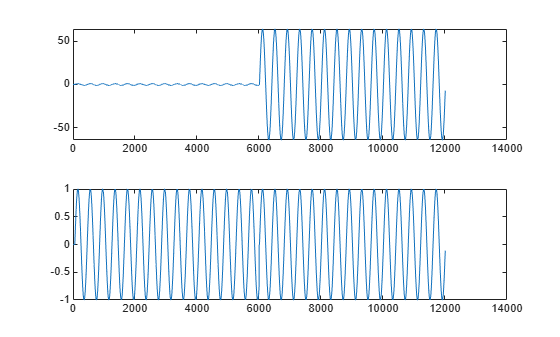# gain

CIC filter gain

## Syntax

```gain(sysobj) gain(sysobj,j) ```

## Description

`gain(sysobj)` returns the gain of the filter System object™ `sysobj`. The function supports the `dsp.CICDecimator` and `dsp.CICInterpolator` filter structures.

When `sysobj` is a decimator, `gain` returns the gain for the overall CIC decimator.

When `sysobj` is an interpolator, the CIC interpolator inserts zeros into the input data stream, reducing the filter overall gain by 1/R, where R is the interpolation factor, to account for the added zero valued samples. Therefore, the gain of a CIC interpolator is (RM)N/R, where N is the number of filter sections and M is the filter differential delay. `gain(sysobj)` returns this value. The next example presents this case.

`gain(sysobj,j)` returns the gain of the `j`th section of a CIC interpolation filter. When you omit `j`, `gain` assumes that `j` is 2*N, where N is the number of sections, and returns the gain of the last section of the filter. This syntax does not apply when `sysobj` is a decimator.

## Examples

collapse all

To compare the performance of two interpolators, one a CIC filter and the other an FIR filter, use gain to adjust the CIC filter output amplitude to match the FIR filter output amplitude. Start by creating an input data set, a sinusoidal signal x.

```fs = 1000; % Input sampling frequency. t = 0:1/fs:1.5; % Signal length = 1501 samples. x = sin(2*pi*10*t); % Amplitude = 1 sinusoid. x = x'; l = 4; % Interpolation factor for FIR filter. d = fdesign.interpolator(l); firInterp = design(d,'multistage','SystemObject',true); yfir = firInterp(x);```
```r = 4; % Interpolation factor for the CIC filter. d = fdesign.interpolator(r,'cic'); cicInterp = design(d,'multisection','SystemObject',true); ycic = cicInterp(x); gaincic = gain(cicInterp); subplot(211); plot([yfir; double(ycic)]); subplot(212) plot([yfir; double(ycic)/gain(cicInterp)]);```After correcting for the gain induced by the CIC interpolator, the second subplot shows that the FIR filter and the CIC filter provide nearly identical interpolation.

This gain equals the gain of the last section of the CIC filter. To confirm, correct the FIR filter amplitude using gain(cicInterp, $2*\mathit{N}$). If $\mathit{N}$ is the number of integrator and comb sections of the CIC filter, then $2*\mathit{N}$ is the last section of the CIC filter.

$\mathit{N}$ is given by cicInterp.NumSections.

The second subplot shows that the FIR filter and CIC filter provide nearly identical interpolation, when the correction gain equals the gain of the last section of the CIC filter.

```subplot(212); plot([yfir; double(ycic)/gain(cicInterp,2*cicInterp.NumSections)]);```Watch now